Try NerdPal! Our new app on iOS and Android

# Simplify the expression $\frac{x^5-x^4+x^2-2}{x^2+1}$

## Related Videos

Go!
Go!
1
2
3
4
5
6
7
8
9
0
a
b
c
d
f
g
m
n
u
v
w
x
y
z
.
(◻)
+
-
×
◻/◻
/
÷
2

e
π
ln
log
log
lim
d/dx
Dx
|◻|
θ
=
>
<
>=
<=
sin
cos
tan
cot
sec
csc

asin
acos
atan
acot
asec
acsc

sinh
cosh
tanh
coth
sech
csch

asinh
acosh
atanh
acoth
asech
acsch

### Videos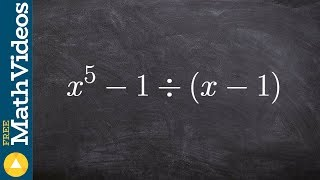### Algebra 2 - Learn how to divide using long division with multiple zeros, (x^5 - 1) / (x - 1)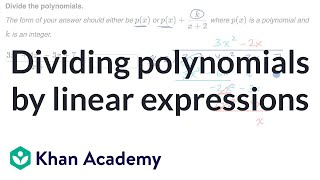### Dividing polynomials by linear expressions | Algebra 2 | Khan Academy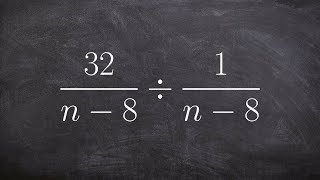### Algebra 2 - Simplify by dividing two rational expressions using factoring (32/n‐8)/(1/n‐8)### Simplifying rational expressions 2 | Polynomial and rational functions | Algebra II | Khan Academy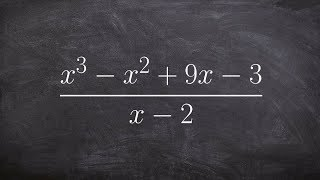### Algebra 2-using synthetic division to divide two polynomials with a remainder (x^3‐x^2+9x‐3)/(x‐2)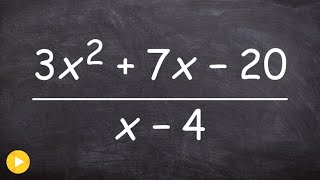SnapXam A2

### beta Got another answer? Verify it!

Go!
1
2
3
4
5
6
7
8
9
0
a
b
c
d
f
g
m
n
u
v
w
x
y
z
.
(◻)
+
-
×
◻/◻
/
÷
2

e
π
ln
log
log
lim
d/dx
Dx
|◻|
θ
=
>
<
>=
<=
sin
cos
tan
cot
sec
csc

asin
acos
atan
acot
asec
acsc

sinh
cosh
tanh
coth
sech
csch

asinh
acosh
atanh
acoth
asech
acsch

$\frac{x^5-x^4+x^2-2}{x^2+1}$